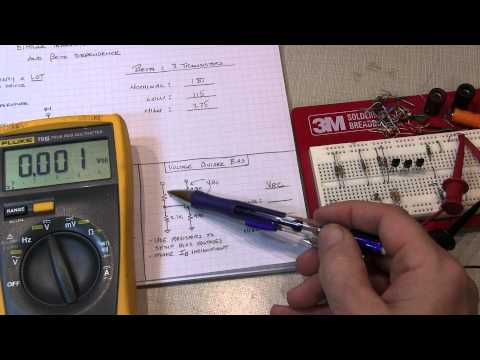# BIPOLAR TRANSISTOR BIAS CIRCUITS AND BETA DEPENDENCE

This tutorial back-to-basics video discusses the operating point (or quiescent point, Q-point, bias point, etc.) of a bipolar transistor (BJT) circuit, and how the choice of the circuit design can affect how sensitive the bias point will be to the value of Beta (current gain) of the transistor.Ideally, you’d want to use a circuit which is completely independent of beta, so that a wide variety of transistors could potentially be used. Three different transistor bias circuits are demonstrated with three transistors, each with a different value of Beta. The current gain of the three transistors are measured and recorded, and then the resulting voltage ACROSS the collector resistors is measured for each transistor in each configuration. In other words the voltage between VCC and the collector is what is measured and recorded (not the collector voltage with respect to ground).

For more detail: BIPOLAR TRANSISTOR BIAS CIRCUITS AND BETA DEPENDENCE